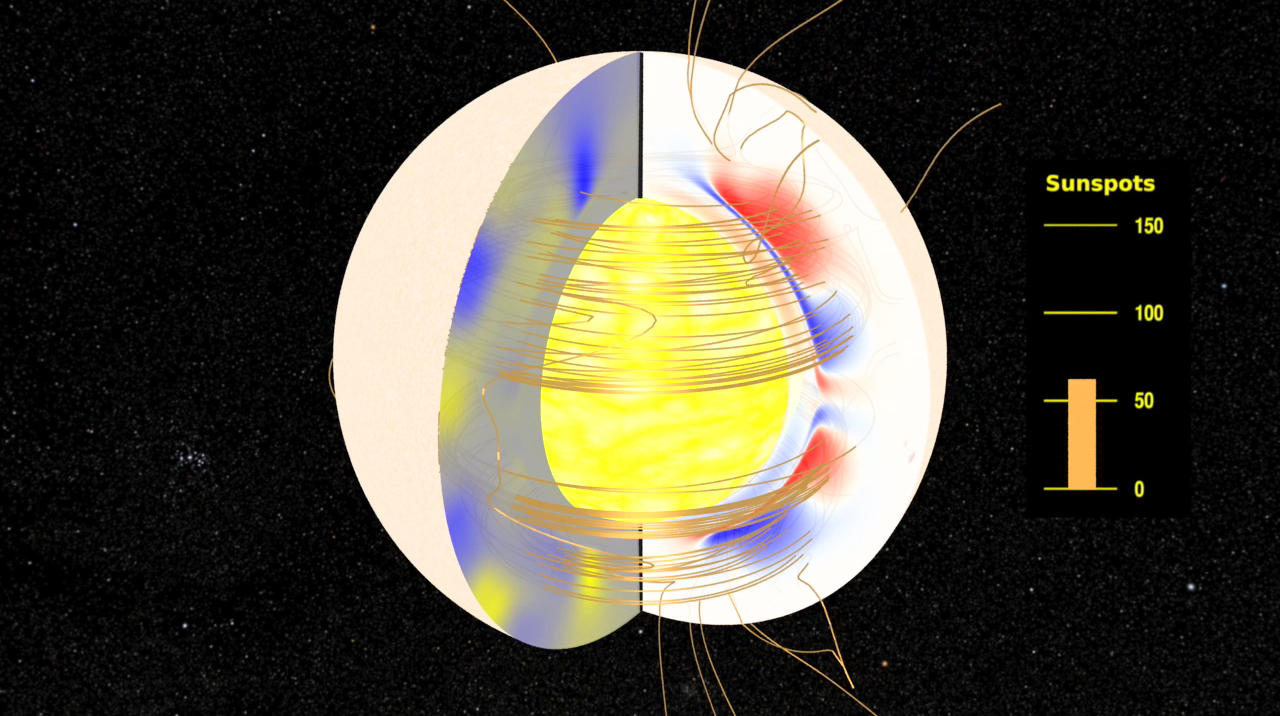## The Solar Dynamo: Toroidal and Radial Magnetic FieldsUsing the solar plasma flows as input (see The Solar Dynamo: Plasma Flows), the equations of magnetohydrodynamics, and 'seeding' the calculations with an initial small magnetic field, one can compute how a magnetic field can grow and be maintained. This is the dynamo process, the net result being that part of the Sun's outflowing thermal convective energy from nuclear processes is used to create the magnetic field.

In this view of the solar dynamo mechanism, we examine the evolution of the toroidal magnetic field, intensities represented by color on the right-hand cross-section, and the radial magnetic field, represented on the left-hand cross-section. To see the poloidal magnetic vector potential, see The Solar Dynamo: Toroidal and Poloidal Magnetic Fields.

In this visualization, the magnetic field lines (represented by the 'copper wire' structures) are 'snapshots' of the field structure constructed at each time step of the model. These field lines should not be considered as 'moving' or 'stretching' as the model evolves in time.

Even this simplified model reproduces a number of characteristics observed in the actual solar magnetic field.

• Cyclic behavior with oscillations in the magnetic field amplitude.
• Magnetic regions at the surface migrate from high latitudes towards the equator. This reproduces the "Butterfly Diagram" pattern.
• Surface magnetic polarities reverse with each cycle

Because this model is axisymmetric, it cannot simulate non-axisymmetric features such as active longitudes.